Ex 5.1

Chapter 5 Class 10 Arithmetic Progressions
Serial order wise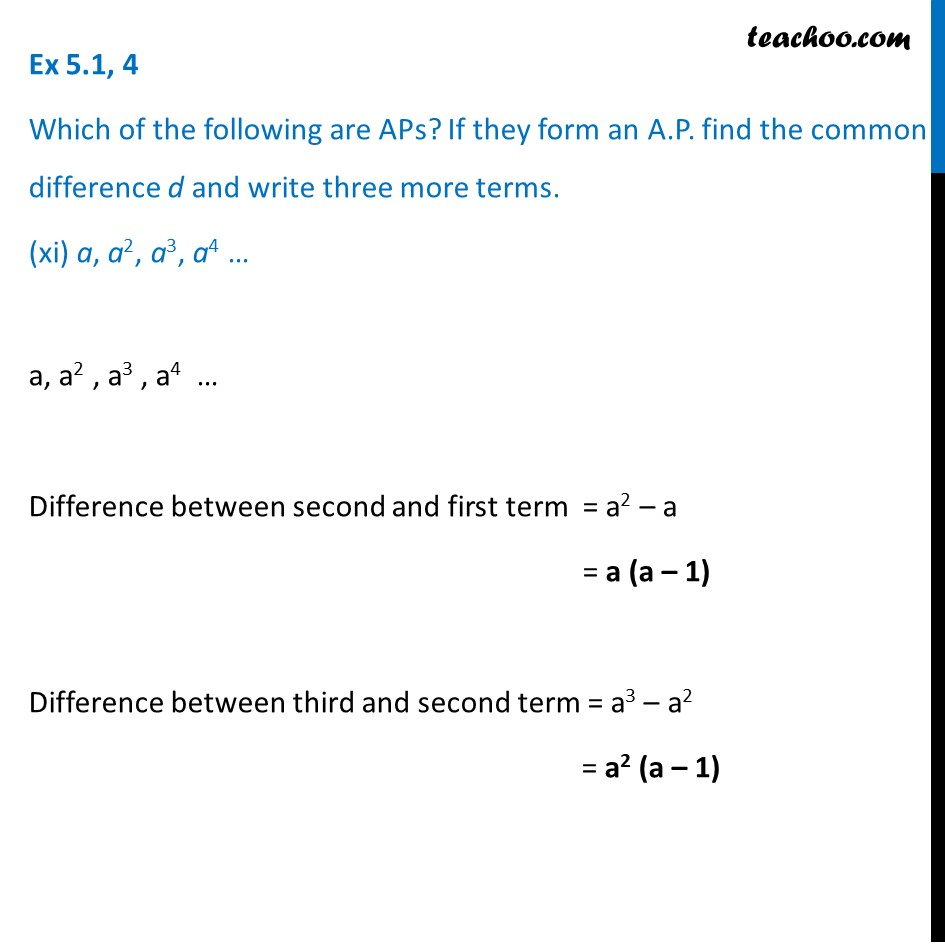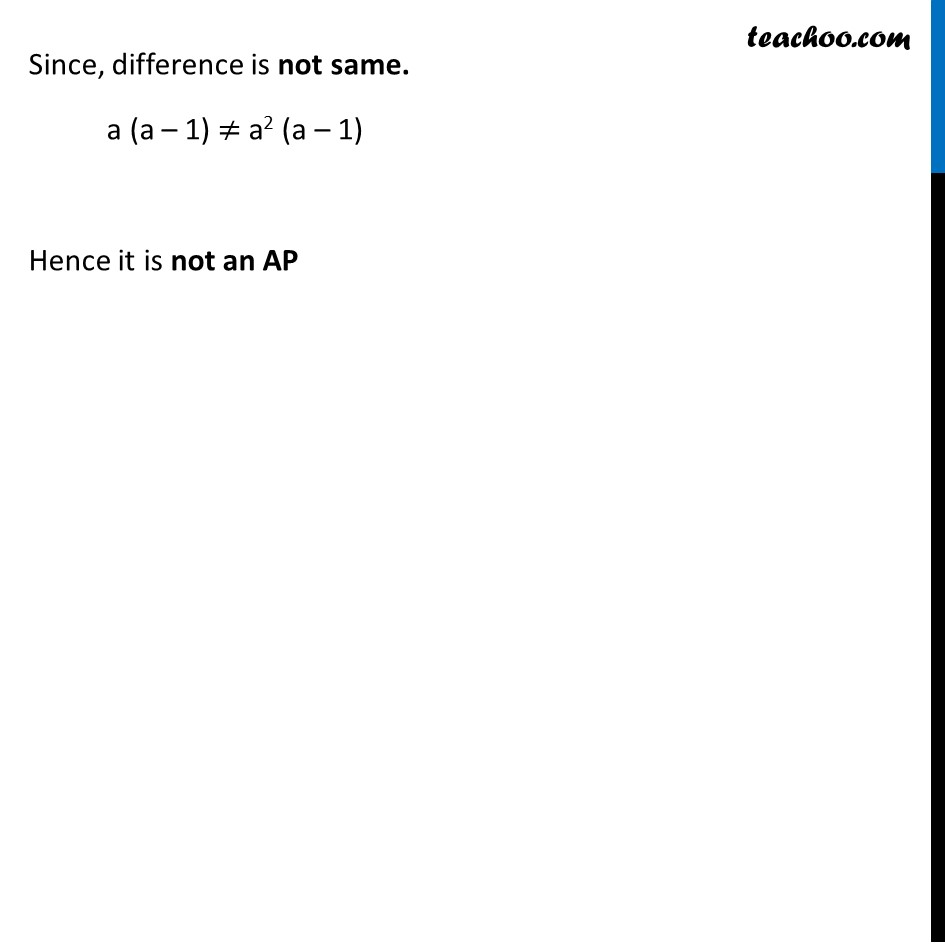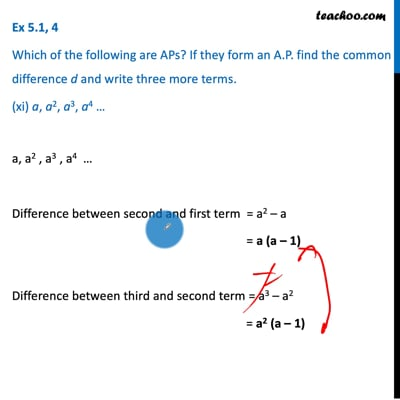This video is only available for Teachoo black users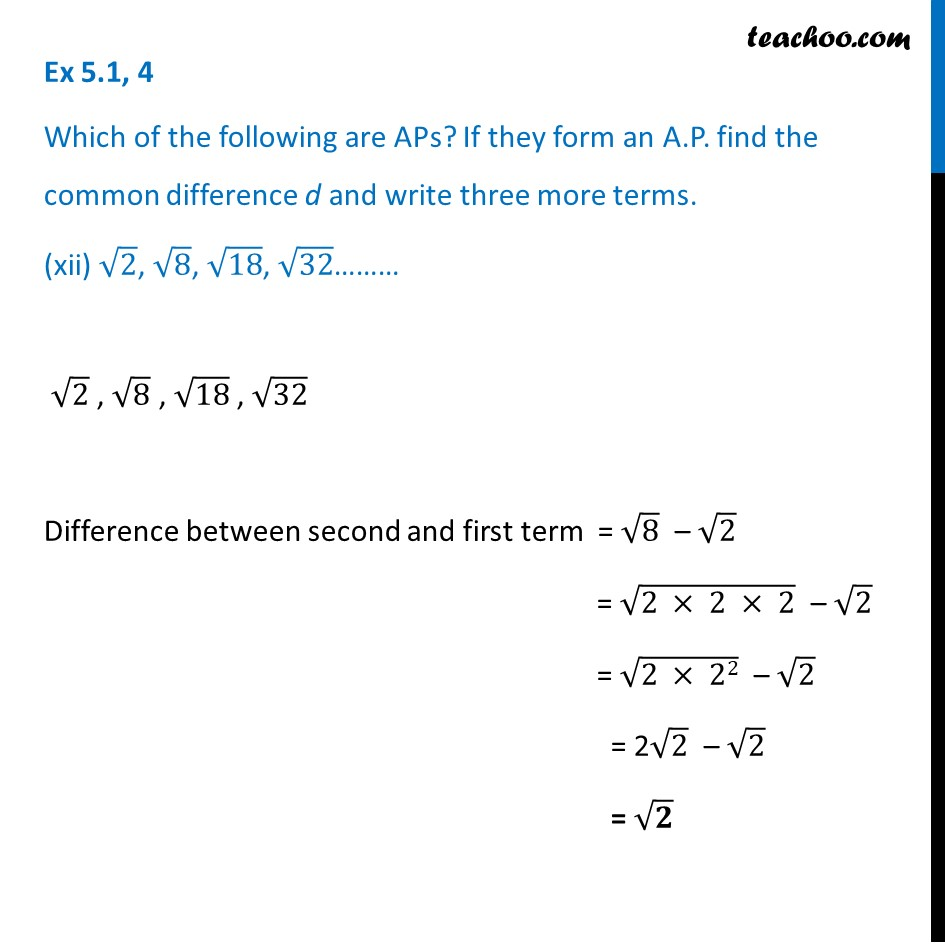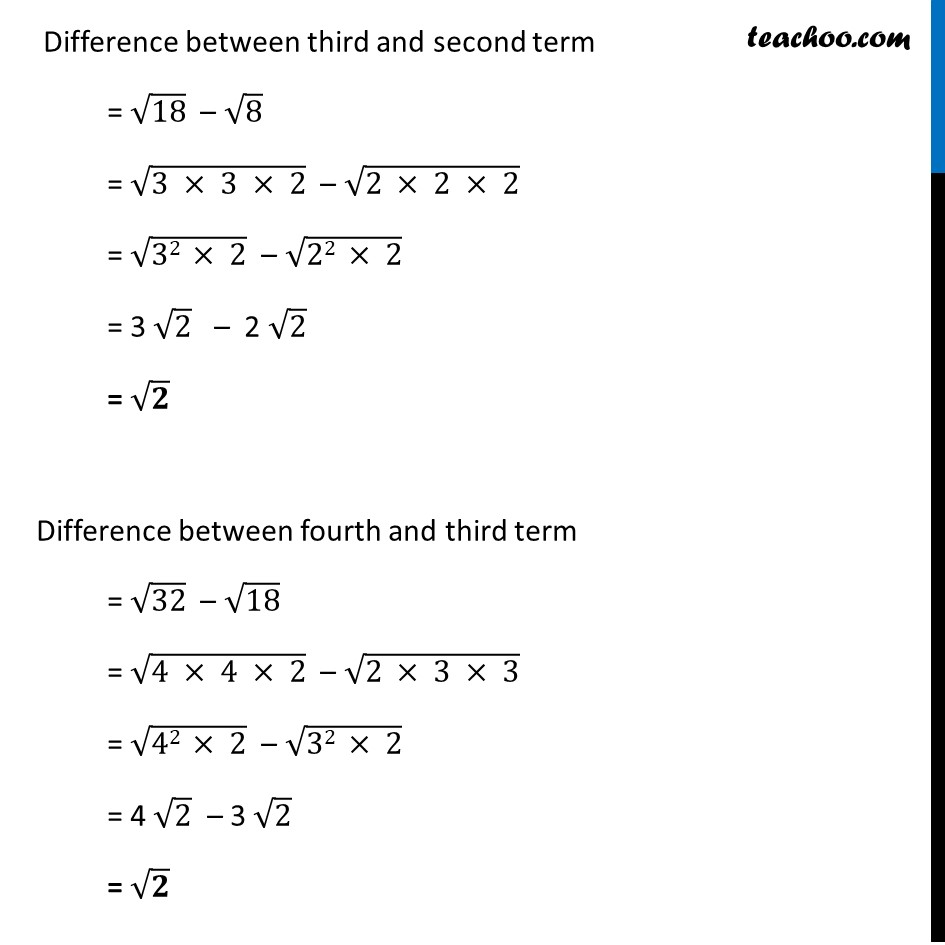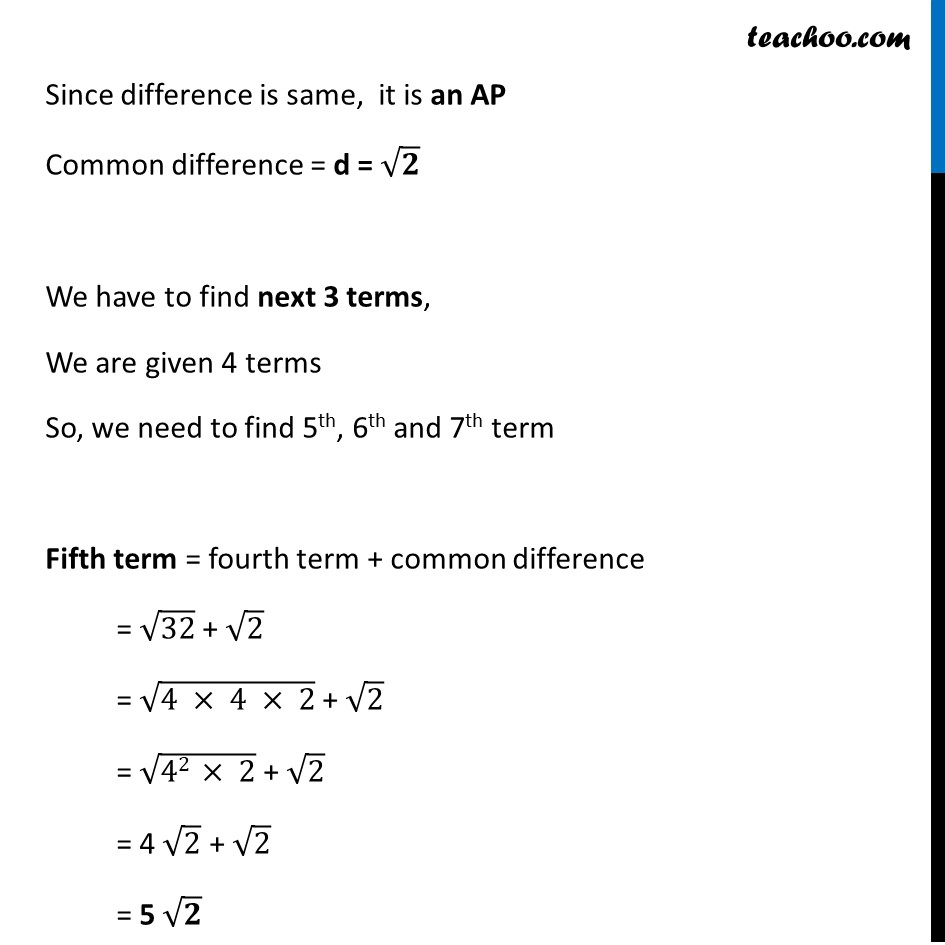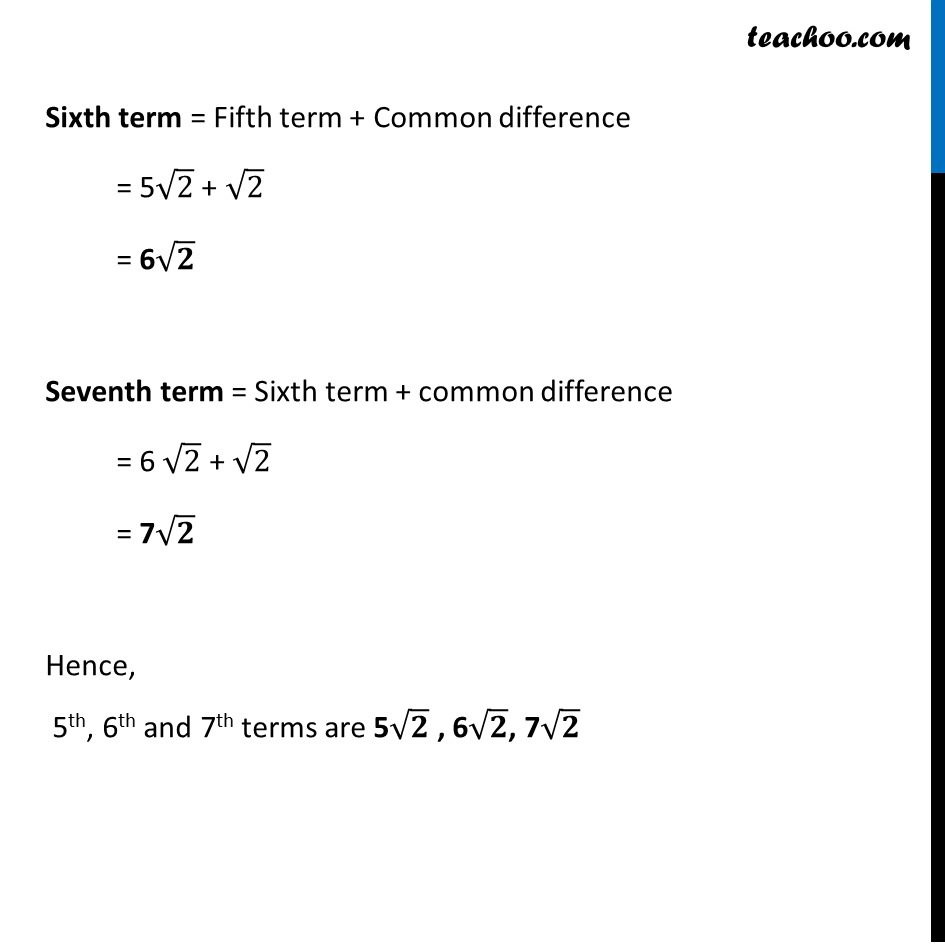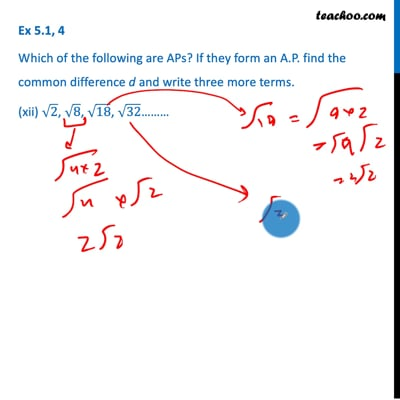This video is only available for Teachoo black users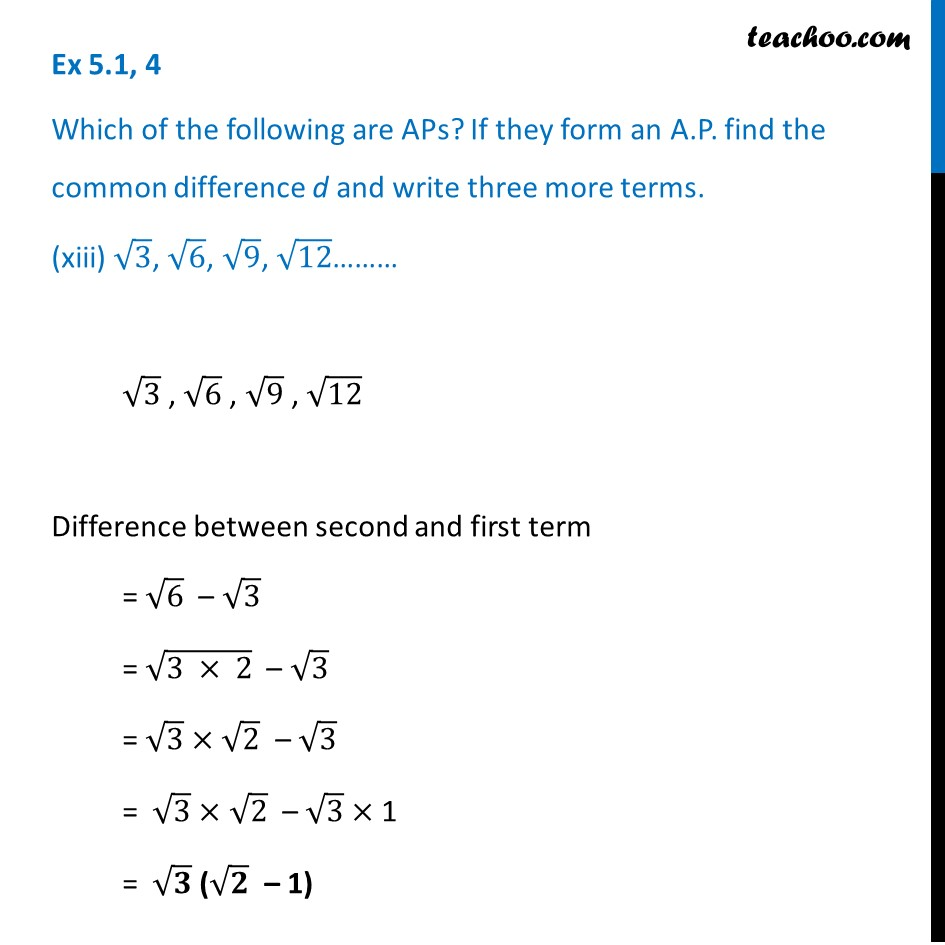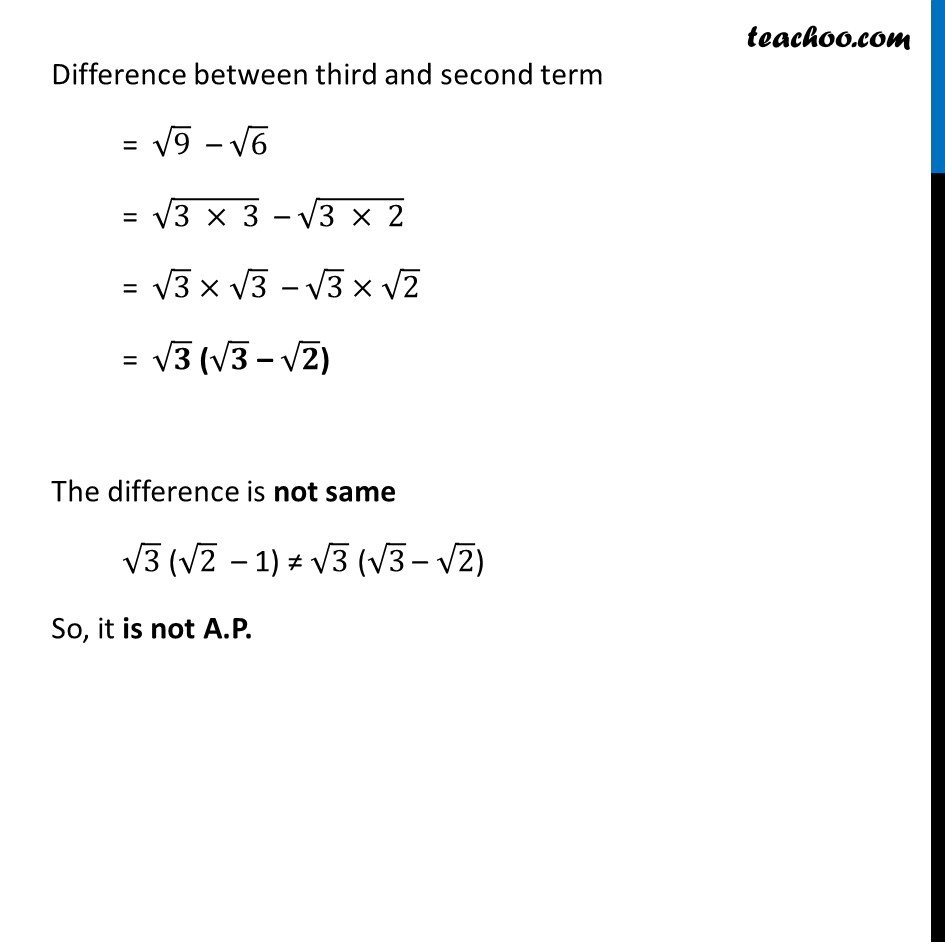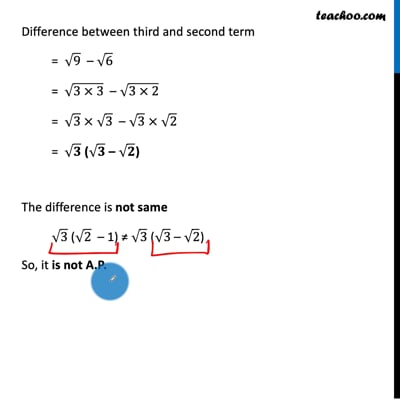This video is only available for Teachoo black users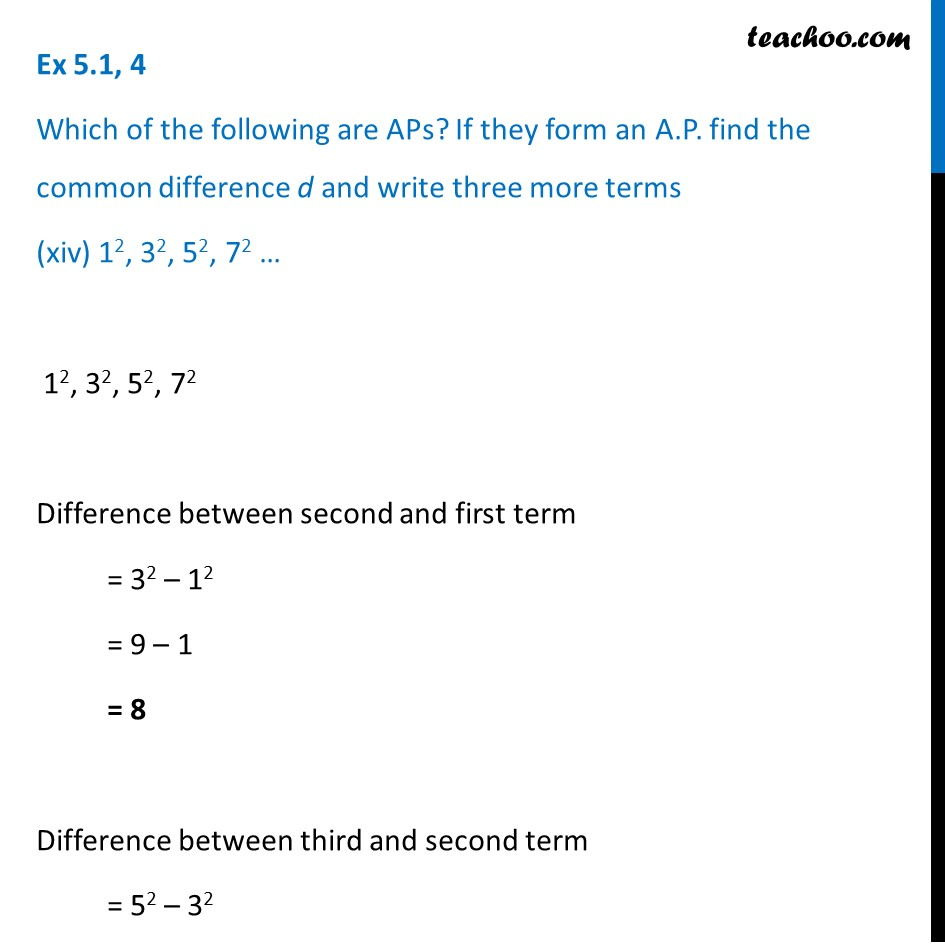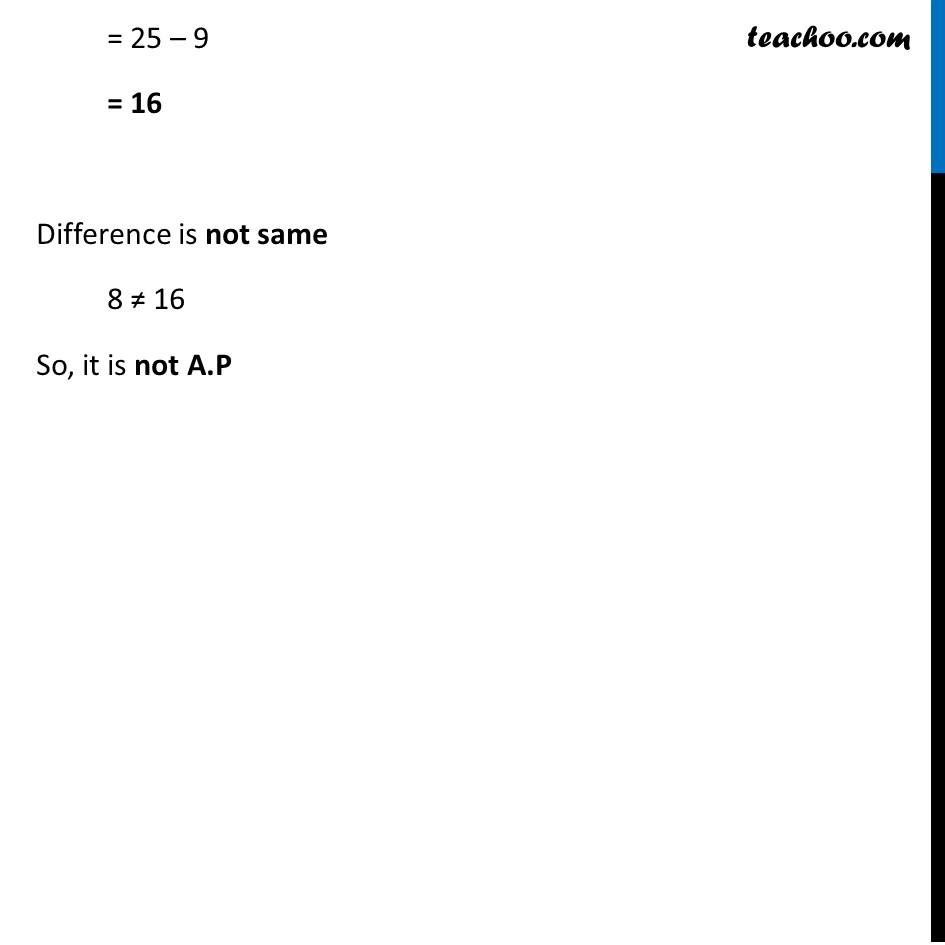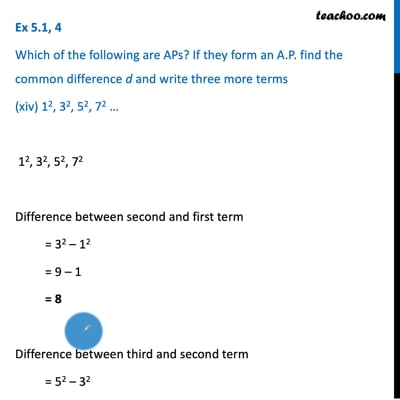This video is only available for Teachoo black users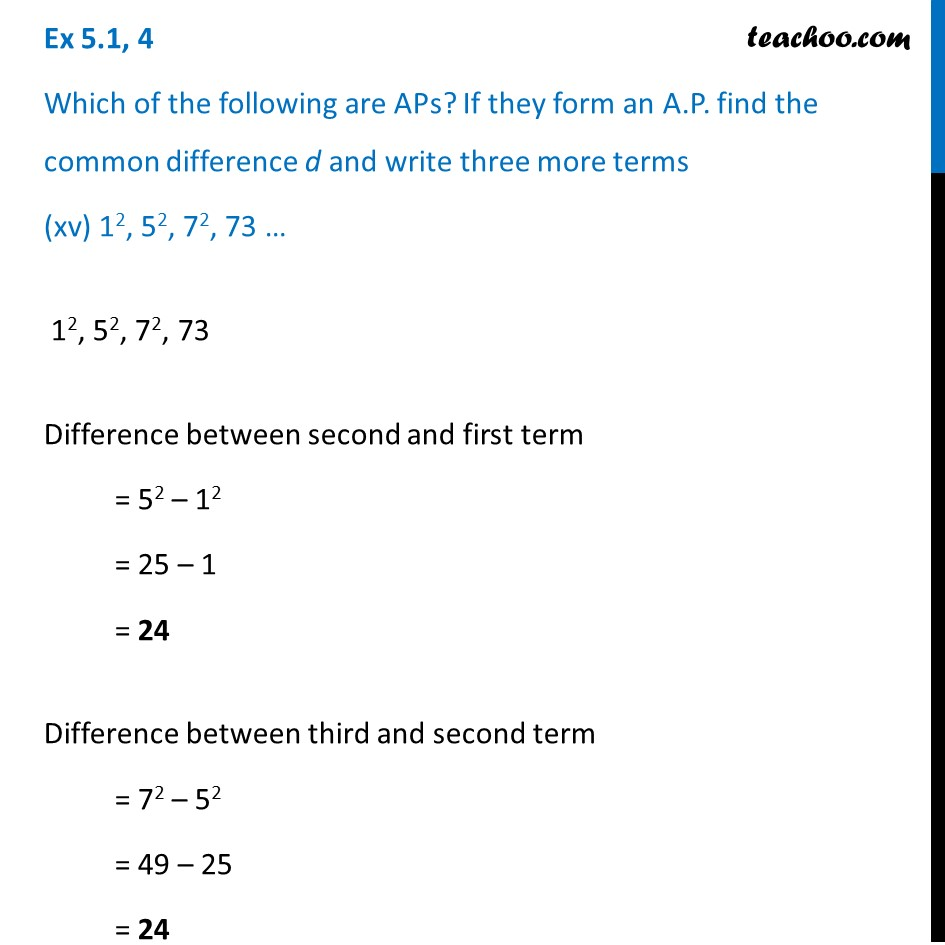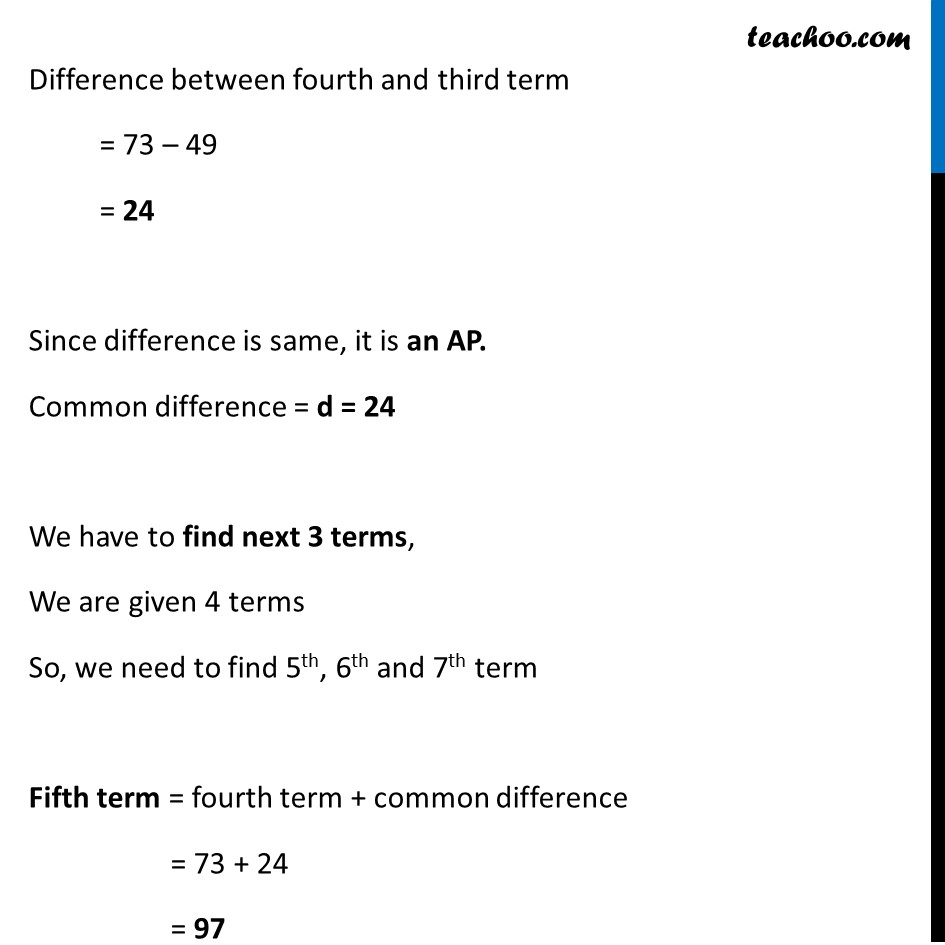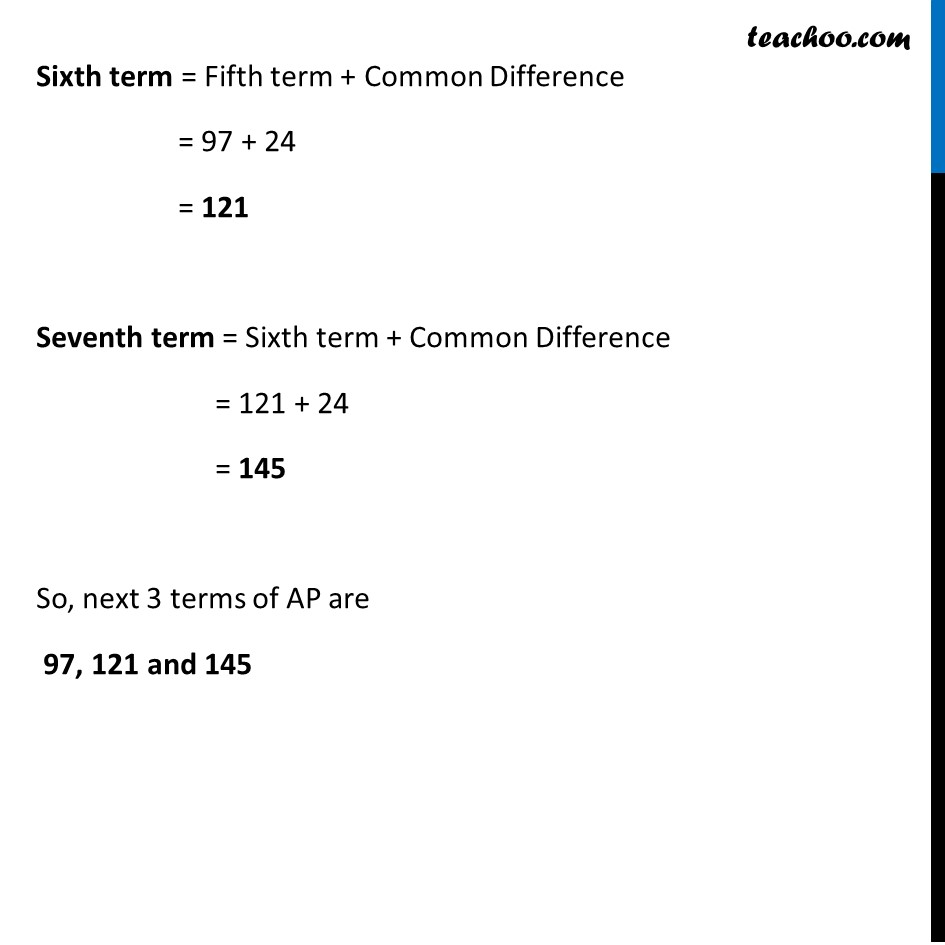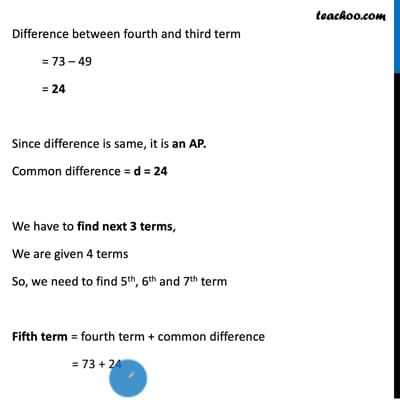This video is only available for Teachoo black users

Solve all your doubts with Teachoo Black (new monthly pack available now!)

### Transcript

Ex 5.1, 4 Which of the following are APs? If they form an A.P. find the common difference d and write three more terms. (xi) a, a2, a3, a4 … a, a2 , a3 , a4 … Difference between second and first term = a2 – a = a (a – 1) Difference between third and second term = a3 – a2 = a2 (a – 1) Since, difference is not same. a (a – 1) ≠ a2 (a – 1) Hence it is not an AP Ex 5.1, 4 Which of the following are APs? If they form an A.P. find the common difference d and write three more terms. (xii) √2, √8, √18, √32……… √2 , √8 , √18 , √32 Difference between second and first term = √8 – √2 = √(2 × 2 × 2) – √2 = √(2 × 22) – √2 = 2√2 – √2 = √𝟐 Difference between third and second term = √18 – √8 = √(3 × 3 × 2) – √(2 × 2 × 2) = √(32 × 2) – √(22 × 2) = 3 √2 – 2 √2 = √𝟐 Difference between fourth and third term = √32 – √18 = √(4 × 4 × 2) – √(2 × 3 × 3) = √(42 × 2) – √(32 × 2) = 4 √2 – 3 √2 = √𝟐 Since difference is same, it is an AP Common difference = d = √𝟐 We have to find next 3 terms, We are given 4 terms So, we need to find 5th, 6th and 7th term Fifth term = fourth term + common difference = √32 + √2 = √(4 × 4 × 2) + √2 = √(42 × 2) + √2 = 4 √2 + √2 = 5 √𝟐 Sixth term = Fifth term + Common difference = 5√2 + √2 = 6√𝟐 Seventh term = Sixth term + common difference = 6 √2 + √2 = 7√𝟐 Hence, 5th, 6th and 7th terms are 5√𝟐 , 6√𝟐, 7√𝟐 Ex 5.1, 4 Which of the following are APs? If they form an A.P. find the common difference d and write three more terms. (xiii) √3, √6, √9, √12……… √3 , √6 , √9 , √12 Difference between second and first term = √6 – √3 = √(3 × 2) – √3 = √3 × √2 – √3 = √3 × √2 – √3 × 1 = √𝟑 (√𝟐 – 1) Difference between third and second term = √9 – √6 = √(3 × 3) – √(3 × 2) = √3 × √3 – √3 × √2 = √𝟑 (√𝟑 – √𝟐) The difference is not same √3 (√2 – 1) ≠ √3 (√3 – √2) So, it is not A.P. Ex 5.1, 4 Which of the following are APs? If they form an A.P. find the common difference d and write three more terms (xiv) 12, 32, 52, 72 … 12, 32, 52, 72 Difference between second and first term = 32 – 12 = 9 – 1 = 8 Difference between third and second term = 52 – 32 = 25 – 9 = 16 Difference is not same 8 ≠ 16 So, it is not A.P Ex 5.1, 4 Which of the following are APs? If they form an A.P. find the common difference d and write three more terms (xv) 12, 52, 72, 73 … 12, 52, 72, 73 Difference between second and first term = 52 – 12 = 25 – 1 = 24 Difference between third and second term = 72 – 52 = 49 – 25 = 24 Difference between fourth and third term = 73 – 49 = 24 Since difference is same, it is an AP. Common difference = d = 24 We have to find next 3 terms, We are given 4 terms So, we need to find 5th, 6th and 7th term Fifth term = fourth term + common difference = 73 + 24 = 97 Sixth term = Fifth term + Common Difference = 97 + 24 = 121 Seventh term = Sixth term + Common Difference = 121 + 24 = 145 So, next 3 terms of AP are 97, 121 and 145